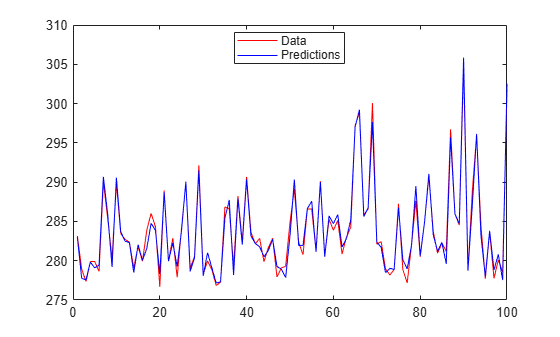# loss

Regression error for Gaussian process regression model

## Syntax

```L = loss(gprMdl,Xnew,Ynew) L = loss(gprMdl,Xnew,Ynew,Name,Value) ```

## Description

`L = loss(gprMdl,Xnew,Ynew)` returns the mean squared error for the Gaussian process regression (GPR) model `gprMdl`, using the predictors in `Xnew` and observed response in `Ynew`.

`L = loss(gprMdl,Xnew,Ynew,Name,Value)` returns the mean squared error for the GPR model, `gprMdl`, with additional options specified by one or more name-value arguments. For example, you can specify a custom loss function or the observation weights.

## Input Arguments

expand all

Gaussian process regression model, specified as a `RegressionGP` (full) or `CompactRegressionGP` (compact) object.

New data, specified as a `table` or an n-by-d matrix, where m is the number of observations, and d is the number of predictor variables in the training data.

If you trained `gprMdl` on a `table`, then `Xnew` must be a `table` that contains all the predictor variables used to train `gprMdl`.

If `Xnew` is a table, then it can also contain `Ynew`. And if it does, then you do not have to specify `Ynew`.

If you trained `gprMdl` on a matrix, then `Xnew` must be a numeric matrix with d columns, and can only contain values for the predictor variables.

Data Types: `single` | `double` | `table`

New observed response values, that correspond to the predictor values in `Xnew`, specified as an n-by-1 vector. n is the number of rows in `Xnew`. Each entry in `Ynew` is the observed response based on the predictor data in the corresponding row of `Xnew`.

If `Xnew` is a table containing new response values, you do not have to specify `Ynew`.

Data Types: `single` | `double`

### Name-Value Arguments

Specify optional comma-separated pairs of `Name,Value` arguments. `Name` is the argument name and `Value` is the corresponding value. `Name` must appear inside quotes. You can specify several name and value pair arguments in any order as `Name1,Value1,...,NameN,ValueN`.

Loss function, specified as `'mse'` (mean squared error) or a function handle.

If you pass a function handle, say `fun`, `loss` calls it as shown below: `fun(Y,Ypred,W)`, where `Y`, `Ypred` and `W` are numeric vectors of length n, and n is the number of rows in `Xnew`. `Y` is the observed response, `Ypred` is the predicted response, and `W` is the observation weights.

Example: `'lossfun',Fct` calls the loss function `Fct`.

Data Types: `char` | `string` | `function_handle`

Since R2023b

Predicted response value to use for observations with missing predictor values, specified as `"median"`, `"mean"`, `"omitted"`, or a numeric scalar.

ValueDescription
`"median"``loss` uses the median of the observed response values in the training data as the predicted response value for observations with missing predictor values.
`"mean"``loss` uses the mean of the observed response values in the training data as the predicted response value for observations with missing predictor values.
`"omitted"``loss` excludes observations with missing predictor values from the loss computation.
Numeric scalar`loss` uses this value as the predicted response value for observations with missing predictor values.

If an observation is missing all predictor values, an observed response value, or an observation weight, then `loss` does not use the observation in the loss computation.

Example: `"PredictionForMissingValue","omitted"`

Data Types: `single` | `double` | `char` | `string`

Observation weights, specified as n-by-1 vector, where n is the number of rows in `Xnew`. By default, the weight of each observation is 1.

Example: `'weights',W` uses the observation weights in vector `W`.

Data Types: `double` | `single`

## Output Arguments

expand all

Regression error for the trained Gaussian process regression model, `gprMdl`, returned as a scalar value.

## Examples

expand all

`load('gprdata.mat')`

The data has 8 predictor variables and contains 500 observations in training data and 100 observations in test data. This is simulated data.

Fit a GPR model using the squared exponential kernel function with separate length scales for each predictor. Standardize the predictor values in the training data. Use the exact method for fitting and prediction.

```gprMdl = fitrgp(Xtrain,ytrain,'FitMethod','exact',... 'PredictMethod','exact','KernelFunction','ardsquaredexponential',... 'Standardize',1);```

Compute the regression error for the test data.

`L = loss(gprMdl,Xtest,ytest)`
```L = 0.6928 ```

Predict the responses for test data.

`ypredtest = predict(gprMdl,Xtest);`

Plot the test response along with the predictions.

```figure; plot(ytest,'r'); hold on; plot(ypredtest,'b'); legend('Data','Predictions','Location','Best');```Manually compute the regression loss.

`L = (ytest - ypredtest)'*(ytest - ypredtest)/length(ytest)`
```L = 0.6928 ```

Load the sample data and store in a `table`.

```load fisheriris tbl = table(meas(:,1),meas(:,2),meas(:,3),meas(:,4),species,... 'VariableNames',{'meas1','meas2','meas3','meas4','species'});```

Fit a GPR model using the first measurement as the response and the other variables as the predictors.

`mdl = fitrgp(tbl,'meas1');`

Predict the responses using the trained model.

`ypred = predict(mdl,tbl);`

Compute the mean absolute error.

```n = height(tbl); y = tbl.meas1; fun = @(y,ypred,w) sum(abs(y-ypred))/n; L = loss(mdl,tbl,'lossfun',fun)```
```L = 0.2345 ```

## Alternatives

You can use `resubLoss` to compute the regression error for the trained GPR model at the observations in the training data.

## Version History

Introduced in R2015b

expand all